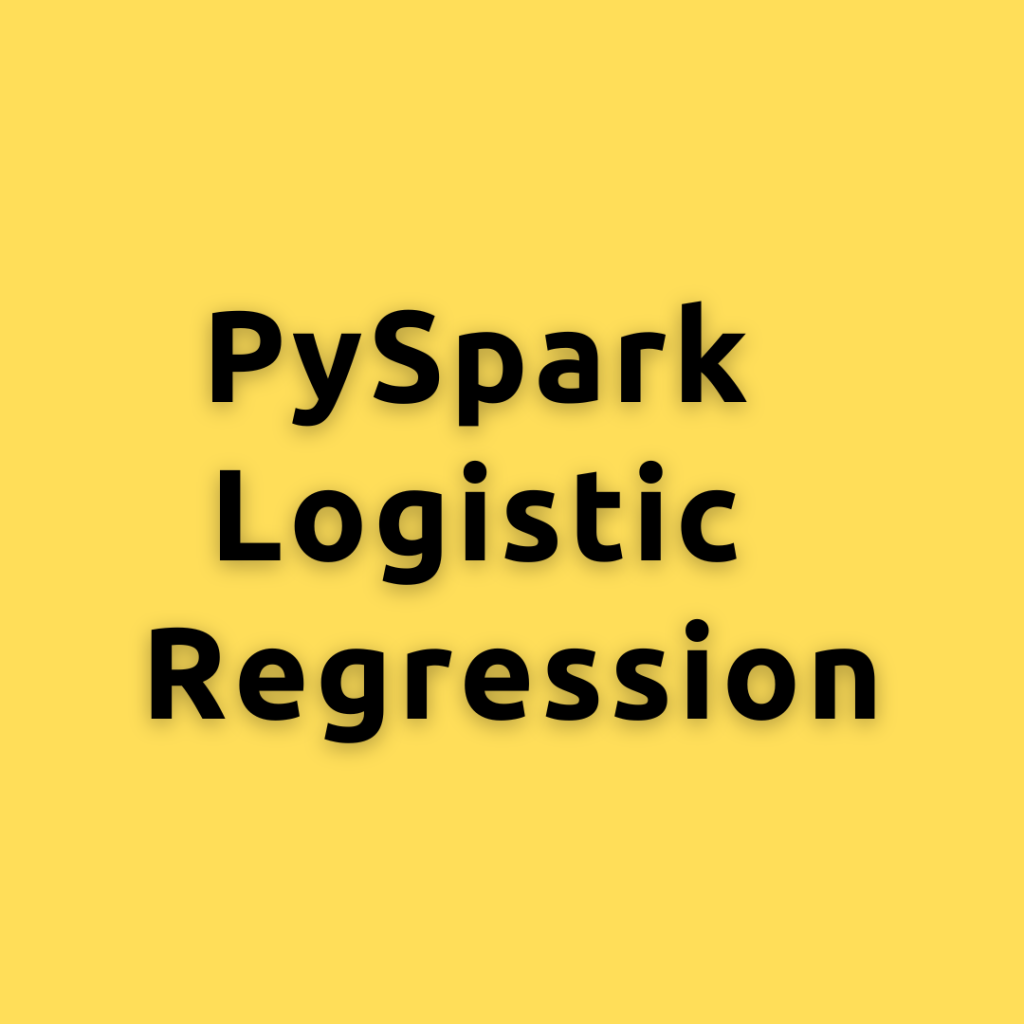Drop a Query

# Logistic Regression## PySpark Logistic Regression – How to Build and Evaluate Logistic Regression Models using PySpark MLlib

Lets explore how to build and evaluate a Logistic Regression model using PySpark MLlib, a library for machine learning in Apache Spark. Logistic Regression is a widely used statistical method for modeling the relationship between a binary outcome and one or more explanatory variables. We will cover the following steps Setting up the environment Loading …## Logistic Regression in Julia – Practical Guide with Examples

Julia is a powerful programming language for Machine Learning and Logistic regression is one of the most popular predictive modeling algorithms, used for binary classification. In this one, you will see the full work flow of how to implement churn modeling using Logistic regression in Julia. Logistic Regression with Julia. Photo by Sergio. This is …Course Preview

## Machine Learning A-Z™: Hands-On Python & R In Data Science

### Free Sample Videos:#### Machine Learning A-Z™: Hands-On Python & R In Data Science#### Machine Learning A-Z™: Hands-On Python & R In Data Science#### Machine Learning A-Z™: Hands-On Python & R In Data Science#### Machine Learning A-Z™: Hands-On Python & R In Data Science